# Algebra 1 : Functions and Lines

## Example Questions

### Example Question #44 : Midpoint Formula

Find the midpoint of the line segment that has endpoints at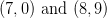.

Possible Answers: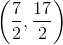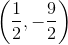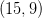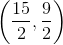Correct answer:Explanation:

To find the midpoint of a line segment, take the average of the x-coordinates and the average of the y-coordinates.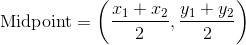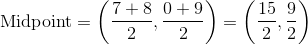### Example Question #45 : Midpoint Formula

Find the midpoint of the line segment that has endpoints at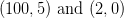.

Possible Answers: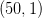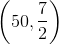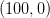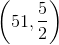Correct answer:Explanation:

To find the midpoint of a line segment, take the average of the x-coordinates and the average of the y-coordinates.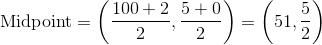### Example Question #46 : Midpoint Formula

Find the midpoint of the line segment that has endpoints at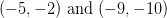.

Possible Answers: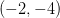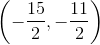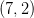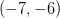Correct answer:Explanation:

To find the midpoint of a line segment, take the average of the x-coordinates and the average of the y-coordinates.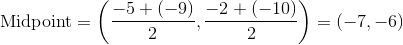### Example Question #47 : Midpoint Formula

Find the midpoint of the line segment that has endpoints at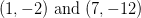.

Possible Answers: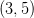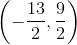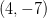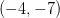Correct answer:Explanation:

To find the midpoint of a line segment, take the average of the x-coordinates and the average of the y-coordinates.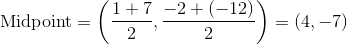### Example Question #48 : Midpoint Formula

Find the midpoint of the line segment that has endpoints at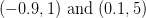.

Possible Answers: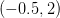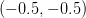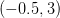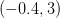Correct answer:Explanation:

To find the midpoint of a line segment, take the average of the x-coordinates and the average of the y-coordinates.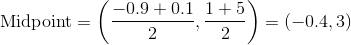### Example Question #49 : Midpoint Formula

Find the midpoint of a line segment that has endpoints at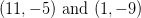.

Possible Answers: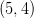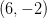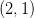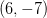Correct answer:Explanation:

To find the midpoint of a line segment, take the average of the x-coordinates and the average of the y-coordinates.### Example Question #41 : How To Find The Midpoint Of A Line Segment

Find the midpoint of a line segment that has endpoints at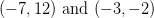.

Possible Answers:Correct answer:Explanation:

To find the midpoint of a line segment, take the average of the x-coordinates and the average of the y-coordinates.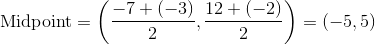### Example Question #42 : How To Find The Midpoint Of A Line Segment

Find the midpoint of the line segment with the endpoints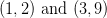.

Possible Answers:Correct answer:Explanation:

To find the midpoint of a line segment, take the average of the x-coordinates and the average of the y-coordinates.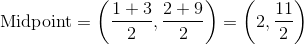### Example Question #43 : How To Find The Midpoint Of A Line Segment

Find the midpoint of the line segment that has endpoints at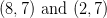.

Possible Answers:Correct answer:Explanation:

To find the midpoint of a line segment, take the average of the x-coordinates and the average of the y-coordinates.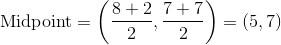### Example Question #44 : How To Find The Midpoint Of A Line Segment

Find the midpoint of the given points: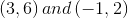Possible Answers:Correct answer:Explanation:

To solve for the midpoint, we want to plug in our coordinate values into the midpoint formula: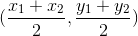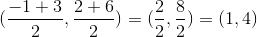### All Algebra 1 Resources